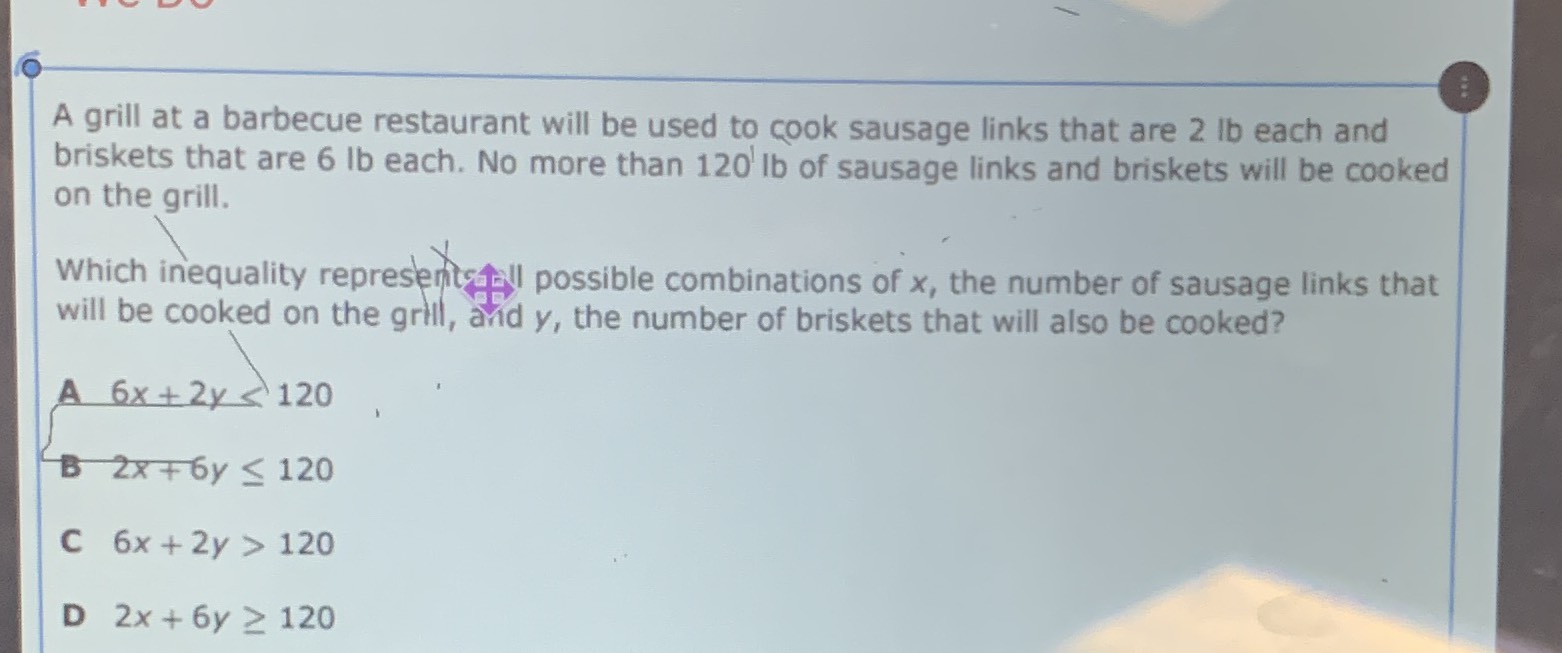### ¿Todavía tienes preguntas de matemáticas?

Pregunte a nuestros tutores expertos
Algebra
PreguntaA grill at a barbecue restaurant will be used to cook sausage links that are $$2 lb$$ each and briskets that are $$6 lb$$ each. No more than $$120 ^ { \prime }$$ lb of sausage links and briskets will be cooked on the grill.

Which inequality representraiall possible combinations of $$x ,$$ the number of sausage links that will be cooked on the grill, arid $$y ,$$ the number of briskets that will also be cooked?

$$6 x + 2 y < 120$$

$$2 x + 6 y \leq 120$$

$$6 x + 2 y > 120$$

$$2 x + 6 y \geq 120$$

$$B)2x+ 6y\le 120$$